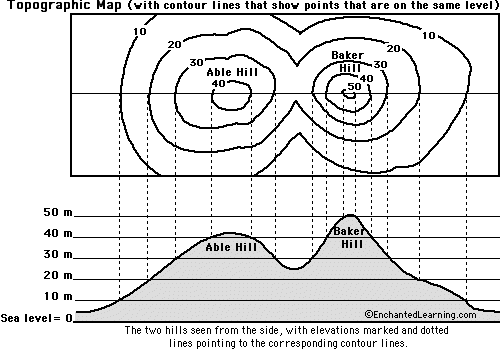1. Hi everyone,

In class, I am given to show that maximizing over x1 first and then maximizing over x2 for f(x1,x2) is the same as maximizing x2 first and then maximizing over x1 for f(x1,x2), assuming f(x1,x2) is always continuous.

Graphically, this statement is apparent to me, because maximizing over x1 first for f(x1,x2) means to find a curve on f(x1,x2) that's only a function of x2 and the maximum point of f(x1,x2) lies on the curve. Maximizing over x2 on this curve means to find the highest point on the curve on the x2 axis. On the other hand, maximizing over x2 first for f(x1,x2) means to find a curve on f(x1,x2) that's only a function of x1 and the maximum point of f(x1,x2) on the curve. Maximizing over x1 on this curve means to find the highest point on the curve on the x1 axis. Either way, the two methods are like the two sides of the same coin in the sense that they both look for the highest point(s) of f(x1,x2).

Algebraically, I'm not sure what my conclusion means. Here's my approach:
(max over x1) (max over x2) f(x1,x2) = (max over x1) g(x1), where x2* = g(x1) is the point of x2 which maximizes f(x1,x2). Then (max over x1) g(x1) = x1* = k for some k in R.
On the other hand,
(max over x2) (max over x1) f(x1,x2) = (max over x2) h(x2), where x1* = h(x2) is the point of x1 which maximizes f(x1,x2). Then (max over x2) h(x2) = x2* = c for some c in R.
Then, in order for (max over x1) (max over x2) f(x1,x2) = (max over x2) (max over x1) f(x1,x2) to be true, the following needs to be true:
g(c) = k
h(k) = c
In particular, this has to be true:
h(g(c)) = c, which implies h = g-1.
I can't make sense of what h = g-1 means graphically or geometrically. I don't even know if I did this right. Could anyone shed any light on this problem and my approach?

Thanks.2.

3. [double entry]4. YES, A ZOMBIE THREAD, but an unanswered one, and one, I think, whose answer would interest others. Consider the topography below ...where, the x axis is the East-West axis, the y axis is the North-South axis, and the z axis is altitude (normal to the screen).

Avle Hill is generally broader though not so tall as Baker Hill, which is generally narrower but overall taller than Able Hill.

If the terrain in maximized in one direction results in the x-z diagram shown below the topo. Maximizing in the other direction gives a y-z diagram of a hill whose height is that of Able Hill in the foothills, but whose height is the peak area of Baker Hill toward the center of the hill. If either resulting diagram is then maximized, it gives the peak of Baker Hill.5. So, from my results, you can see wj_green's thinking in reaching his first conclusion: Regardless of which axis is maximized first, as long as both aces are maximized, the answer is always max z.

However, wj_green explores further and, sadly, I quickly become lost. If anyone wants to take over for me, I very gladly welcome them to it. I think wj_green is saying that there isn't any obvious relationship between the locations that have equal heights, For example, the location on the eastern foothlls of Baker Hill in the x-z diagram is the same height (let's use 20 meters as an example) as the locations in the northern and southern foothills of Able Hill in the y-z diagram. But apparently, there's no relationship between these locations. which is true, I think this is obvious from the diagrams I have posted and designed.Bookmarks
 Posting Permissions
 You may not post new threads You may not post replies You may not post attachments You may not edit your posts   BB code is On Smilies are On [IMG] code is On [VIDEO] code is On HTML code is Off Trackbacks are Off Pingbacks are Off Refbacks are On Terms of Use Agreement